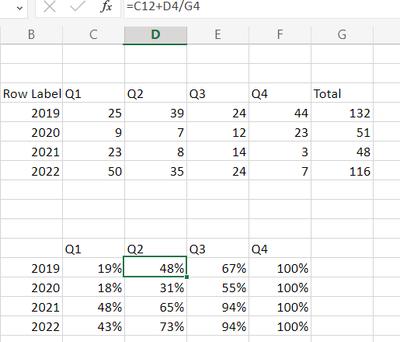## cumulative percentages

Hi,

I have a dataset where I created a table in Excel that looks like the first example below. That shows, for example that in 2019, number of records for Q1 was 25, and son on. I calculated the Total with a very basic formula. It is easy to replicate it in VA using crosstab. However, I am struggling it to turn it into the second example. My question is, how to calculate Total for each year and then use this number to calculate the percentages? The other tricky part is that the percentages are cumulative in the second example.

Thank you!Sam_SAS
SAS Super FREQ

## Re: cumulative percentages

Hello,

I believe you could calculate this by using two of the periodic operators in VA:

CumulativePeriod will return the year-to-date value for each quarter if you specify ByQuarter as the inner interval and ByYear as the outer interval.

Period will return the value for the current year if you specify ByYear as the interval.

So, you could make an expression like this:

```CumulativePeriod(_Sum_, 'Expenses'n, _IgnoreAllTimeFrameFilters_,
'Quarter'n, _ByQuarter_, _ByYear_, 0, 1, _Full_, {Date}) / Period(_Sum_, 'Expenses'n, _IgnoreAllTimeFrameFilters_, 'Quarter'n,
_ByYear_)```

And get a result like this:This is not exactly the same thing as the data in your opening post. You need the quarter and year to be the same column for this to work. In addition, your report object can only have one date column it for the periodic functions to work. You could work around this somewhat by making an extra character column with the years, for example.

Let us know if that helps,

Sam

Discussion stats